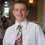# Sum of Multichoose Reciprocals

I stumbled across an interesting formula involving the multichoose function. Let $m,n$ be natural numbers with $m > 1$, and suppose we want to find the sum of the reciprocals of the first $n$ multichoose coefficients. After experimenting a little, I found this surprisingly elegant formula:

$\sum_{r = 1}^{n} \left(\!\!{r\choose m}\!\!\right)^{-1} = \dfrac{m}{m-1} \left(1 - \left(\!\!{m\choose n}\!\!\right)^{-1} \right)$

Furthermore, if we add them to infinity, the formula simplifies to $\sum_{r = 1}^{\infty} \left(\!\!{r\choose m}\!\!\right)^{-1} = \dfrac{m}{m-1}$

So my question is: can you think of a nice combinatorial proof of this formula (without using induction)?

For those of you who don't know, $\left(\!\!{n\choose m}\!\!\right)$ denotes the number of different ways to pick a bunch of $m$ smarties with $n$ distinct choices of colour, where order is not important but repetition of colours is allowed.

It can also be defined this way: $\left(\!\!{n\choose m}\!\!\right) = \binom{n+m-1}{m}$Note by Ariel Gershon
6 years, 6 months ago

This discussion board is a place to discuss our Daily Challenges and the math and science related to those challenges. Explanations are more than just a solution — they should explain the steps and thinking strategies that you used to obtain the solution. Comments should further the discussion of math and science.

When posting on Brilliant:

• Use the emojis to react to an explanation, whether you're congratulating a job well done , or just really confused .
• Ask specific questions about the challenge or the steps in somebody's explanation. Well-posed questions can add a lot to the discussion, but posting "I don't understand!" doesn't help anyone.
• Try to contribute something new to the discussion, whether it is an extension, generalization or other idea related to the challenge.

MarkdownAppears as
*italics* or _italics_ italics
**bold** or __bold__ bold
- bulleted- list
• bulleted
• list
1. numbered2. list
1. numbered
2. list
Note: you must add a full line of space before and after lists for them to show up correctly
paragraph 1paragraph 2

paragraph 1

paragraph 2

[example link](https://brilliant.org)example link
> This is a quote
This is a quote
    # I indented these lines
# 4 spaces, and now they show
# up as a code block.

print "hello world"
# I indented these lines
# 4 spaces, and now they show
# up as a code block.

print "hello world"
MathAppears as
Remember to wrap math in $$ ... $$ or $ ... $ to ensure proper formatting.
2 \times 3 $2 \times 3$
2^{34} $2^{34}$
a_{i-1} $a_{i-1}$
\frac{2}{3} $\frac{2}{3}$
\sqrt{2} $\sqrt{2}$
\sum_{i=1}^3 $\sum_{i=1}^3$
\sin \theta $\sin \theta$
\boxed{123} $\boxed{123}$

Sort by:

$\sum_{r = 1}^{\infty} \left(\!\!{r\choose m}\!\!\right)^{-1} = \dfrac{m}{m-1}$

$\displaystyle \left(\!\!{r \choose m}\!\!\right) = \binom{r+m - 1}{m}$

$\displaystyle \sum_{r = 1}^{\infty} \dfrac{1}{\binom{r+m - 1}{m}} = \dfrac{(r - 1)!\times m!}{(m+r - 1)!}$

$= \dfrac{m!}{(r)(r+1).......(m + r - 1)}$

$\dfrac{1}{(r)(m + r - 1)} = \dfrac{1}{m - 1} \left ( \dfrac{1}{r} - \dfrac{1}{m + r - 1} \right)$

$\dfrac{m!}{(r)(r+1).......(m + r - 1)} = \dfrac{m!}{m - 1}\left( \dfrac{1}{r(r+1)....(m+r-2)} - \dfrac{1}{(r+1)....(m + r - 1)}\right)$

$\dfrac{m!}{m - 1} \times \dfrac{1}{(m - 1)!} = \dfrac{m}{m - 1}$

- 6 years, 6 months ago

Yes, that's the algebraic proof using telescoping series, which is in essence a form of induction.

Is there any other way of interpreting this formula? For example, if we could write it as a taylor series expansion?

A combinatorial proof seems less likely to me, because there is no clear interpretation of $\left( {r \choose m } \right)$, though I might be wrong.

Staff - 6 years, 6 months ago

Perhaps you're right that there's no good combinatorial proof. However the interpretation of $\left(\!\!{n \choose m}\!\!\right)$ is the number of different multisets of size $m$ (where order is not important but repetition is allowed) which contain elements chosen from $\{1,2,3, ... n\}$.

We can always convert the formula into one that only involves binomial coefficients: $\sum_{k = m}^n \dfrac{1}{{k\choose m}} = \dfrac{m}{m-1} \left(1 - \dfrac{1}{\binom{n}{m-1}}\right)$

- 6 years, 6 months ago

Hi guys I wish to improve my mathematics skill , so can anyone help me with it , how do I proceed with this idea ?

- 6 years, 6 months ago

Check out the wikis (in the practice section), and work on more problems!

Staff - 6 years, 6 months ago